# Experience The Math Translator Difference

The Math Translator offers comprehensive instructional video support for the OpenStax books ranging from Prealgebra to Precalculus.  If you're a teacher/professor, these videos can be used as a lecture source in your online or hybrid courses or as a supplement to your classroom instruction. If you're homeschooling, this service can be used as a complete math resource for your children. In addition to the video instruction on this site, you will find answer keys for the practice tests in each chapter of each book.

### About the Textbooks Experts in their Field

The Math Translator video library provides companion instruction to the OpenStax™ math textbooks.  OpenStax is a nonprofit educational initiative based at Rice University. The authors of the OpenStax textbooks are content experts in their field. The textbooks are peer-reviewed, openly licensed, 100% free, and available online. The OpenStax's mission is to “improve educational access and learning for everyone”.### About the VideosCreated by a Tenured Math Professor

My mission as The Math Translator is in direct alignment with the OpenStax mission and The Math Translator is an OpenStax Partner Ally. I believe strongly in the value and importance of education and I know that high-quality instruction is a crucial component of learning mathematics. My videos provide companion instruction to the OpenStax math textbooks. Every section of every book has a comprehensive 1-hour lecture video that teaches that section's learning objectives in depth. In addition to this, I have recorded video explanations for every other odd homework problem in each book. The Math Translator provides the most comprehensive instructional resource available for the OpenStax math textbooks.

### About the Video LibraryComprehensive Instruction to Accompany each OpenStax Math Textbook

Each video library is composed of three elements: comprehensive video lectures, homework support videos, and written answer keys for each practice test in the book.  The video library outlines give an overview of the content of each lecture as well as suggested homework exercises.  The answer keys for the Chapter 1 Practice Tests have been posted here; for the rest of the answer keys, please login.

•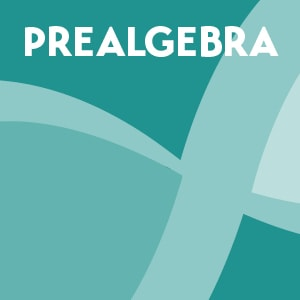#### Prealgebra

This video library accompanies the OpenStax Prealgebra 2e. textbook.  The videos cover the fundamental arithmetic and geometry skills needed for success in algebra.  Topics include whole numbers, the language of algebra, integers, fractions, decimals, percents, the properties of real numbers, solving linear equations, math models and geometry, polynomials, and graphs. This video library contains 62 comprehensive 1-hour lectures and 963 homework support videos, in addition to answer keys for each practice test in the book.

Video Library Outline

Chapter 1 - Practice Test Answer Key.pdf

•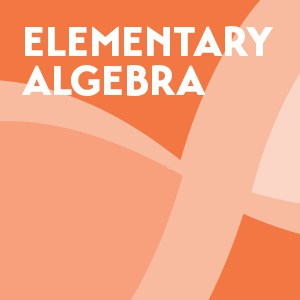#### Elementary Algebra

This video library accompanies the OpenStax Elementary Algebra 2e. textbook. The videos cover the fundamental concepts of algebra.  Topics include solving linear equations and inequalities, math models, linear graphs, systems of linear equations, polynomials, factoring, rational expressions and equations, roots and radicals, and quadratic equations. The video library contains 80 comprehensive 1-hour lectures and 1,116 homework support videos, in addition to answer keys for each practice test in the book.

Video Library Outline

Chapter 1 - Practice Test Answer Key.pdf

•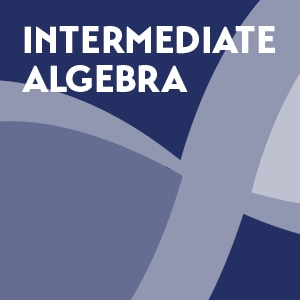#### Intermediate Algebra

This video library accompanies the OpenStax Intermediate Algebra 2e. textbook. The videos cover algebra concepts in the depth needed to prepare students for college algebra.  Topics include solving linear equations, graphs and functions, systems of linear equations, polynomials and polynomial functions, factoring, rational expressions and functions, roots and radicals, quadratic equations and functions, exponential and logarithmic functions, conic sections, and series, sequences, and the binomial theorem. The video library contains 95 comprehensive 1-hour lectures and 1,021 homework support videos, in addition to answer keys for each practice test in the book.

Video Library Outline

Chapter 1 - Practice Test Answer Key.pdf

•#### College Algebra

This video library accompanies the OpenStax College Algebra 2e. textbook. The videos provide a comprehensive exploration of algebra principles. Topics include equations and inequalities, functions, linear functions, polynomial and rational functions, exponential and logarithmic functions, systems of equations and inequalities, analytic geometry, sequences, probability, and counting theory. The video library contains 65 comprehensive 1-hour lectures and 730 homework support videos, in addition to answer keys for each practice test in the book.

Video Library Outline

Chapter 1 - Practice Test Answer Key.pdf

•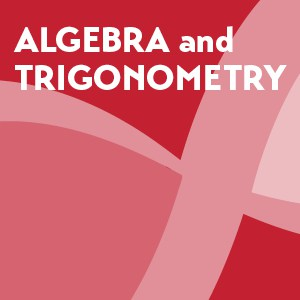#### Algebra and Trigonometry

This video library accompanies the OpenStax Algebra and Trigonometry 2e. textbook.  The videos provide in depth coverage of algebra and trigonometry principles. Topics include equations and inequalities, functions, linear functions, polynomial and rational functions, exponential and logarithmic functions, the unit circle, periodic functions, trigonometric identities and equations, further applications of trigonometry, systems of equations and inequalities, analytic geometry, sequences, probability, and counting theory. This video library contains 85 comprehensive 1-hour lectures and 974 homework support videos, in addition to answer keys for each practice test in the book.

Video Library Outline

Chapter 1 - Practice Test Answer Key.pdf

•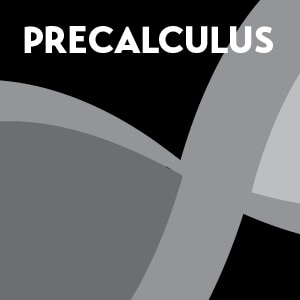#### Precalculus

This video library accompanies the OpenStax Precalculus 2e. textbook.  The videos cover the algebra and trigonometry principles needed for success in calculus. Topics include functions, linear functions, polynomial and rational functions, exponential and logarithmic functions, trigonometric functions, periodic functions, trigonometric identities and equations, further applications of trigonometry, systems of equations and inequalities, analytic geometry, and sequences, probability, and counting theory. This video library contains 73 comprehensive 1-hour lectures and 800 homework support videos, in addition to answer keys for each practice test in the book.

Video Library Outline

Chapter 1 - Practice Test Answer Key.pdf

### Homeschool Information

The Math Translator videos can be used as a stand alone math curriculum from Prealgebra to Precalculus for homeschooled children.  To learn more about how to use The Math Translator to homeschool your child(ren) in math, click here.